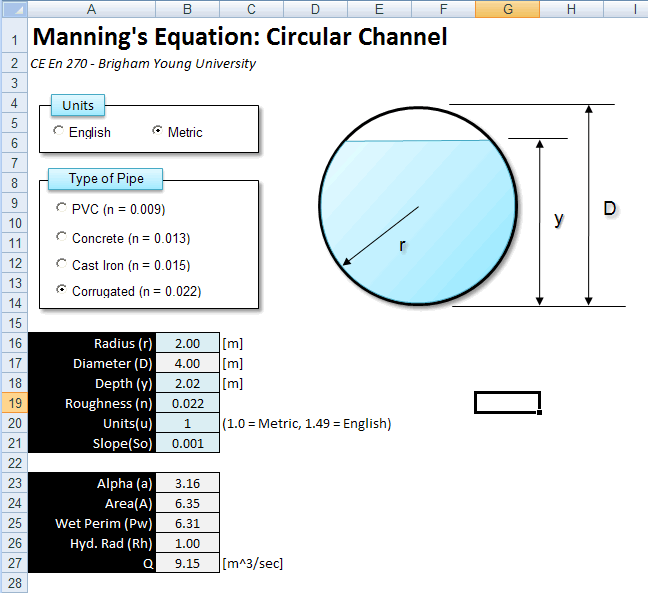# HW#14

## Part A - Debugging

Click here to download an Excel spreadsheet with VB code for computing attributes of a triangle using the law of sines and the law of cosines.  Each of the four buttons has associated VB code to perform the indicated calculations.  Each of the four subroutines has at least one bug.  Use the debugger to find and fix the subroutines.  The following sets of correct answers will help you determine when the code is giving you the proper results:

Law of Sines - Side B

Side A: 8
Angle A: 31
Angle B: 30
Side B = 7.77

Law of Sines - Angle B

Side A: 8
Side B: 5
Angle A: 31
Angle B = 18.78

Law of Cosines - Angle C

Side A: 120
Side B: 140
Side C: 75
Angle C = 32.38

Law of Cosines - Side C

Side A: 120
Side B: 140
Angle C: 35
Side C = 80.48

## Part B - Manning's Equation on a Circular Channel

In Homework #10, you added a set of option controls to a spreadsheet that solves Manning's Equation for a circular channel.  The spreadsheet looked something like this:Modify your spreadsheet so that it uses a combo box to select the Type of Pipe rather than a set of option controls.  Put the choices for the combo box on a second page.  Modify the code so that the roughness value in cell B19 is updated whenever the user makes a new selection with the combo box.